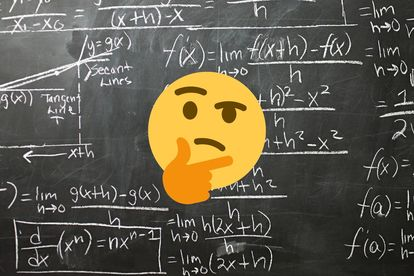This maths equation has left boffins divided over the correct process for solving it, with two methods resulting in two different answers. Photo: Canva

# Can you solve this maths equation that has the internet baffled?

This maths equation has left boffins divided over the correct process for solving it, with two methods resulting in two different answers.This maths equation has left boffins divided over the correct process for solving it, with two methods resulting in two different answers. Photo: Canva

The ‘basic’ maths equation below has left boffins divided over the correct process for solving it, with two methods resulting in two completely different answers.

ALSO READ | Are you smarter than a matric student: Can you answer these maths questions?

Is the answer ONE or NINE – or perhaps something else?

Those South Africans who can still remember back to their school days, will not-so-fondly recall being taught ‘BODMAS’ when attempting to solve such problems.

BODMAS is an acronym to help pupils remember the correct order in which to solve maths problems.

ALSO READ | SOUTH AFRICA’S TOP PUPIL PLANS TO STUDY AT UCT

A quick reminder, it stands for for B-Brackets, O-Orders (powers/indices or roots), D-Division, M-Multiplication, A-Addition, S-Subtraction.

Using the above, the answer would appear to be ONE.

MUST READ | MATRIC RESULTS 2022: TOP 9 PRIVATE BOYS SCHOOLS IN SOUTH AFRICA

However!

Following today’s standards, the process for solving the equation is to address what is in the brackets first.

So, 1+2 becomes 3, and the equation becomes 6 ÷ 2 (3).

The brackets around the 3 indicates it is to be multiplied by what comes before it, so the equation translates to 6 ÷ 2 x 3.

As multiplication and division are of the same precedence, the equation is then solved left to right: 6 ÷ 2 becomes 3, making the equation 3 x 3, resulting in a solution of NINE.

MUST READ | MEET THE TOP STUDENT IN SOUTH AFRICA!

What answer do YOU get? Let us know by clicking on the ‘comment’ tab below this article.